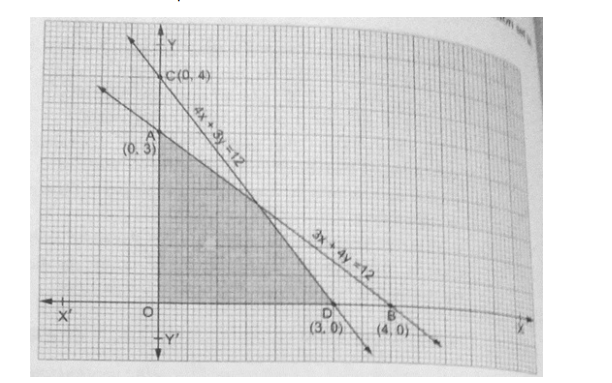# Find the linear inequalities for which the shaded area is the solution set in the figure given below.

Question:

Find the linear inequalities for which the shaded area is the solution set in the figure given below.Solution:

We have seen that the shaded region and origin are on the same side of the line $3 x+4 y=12$

For $(0,0)$ we have $0+0-12<0$. So the shaded region satisfies the inequality $3 x+4 y \leq 12$.

We have seen that the shaded region and origin are on the same side of the line $4 x+3 y=12$

For $(0,0)$ we have $0+0-12<0$. So the shaded region satisfies the inequality $4 x+3 y \leq 12$.

Also, the region lies in the first quadrent. Therefore $x \geq 0$

and $y \geq 0$

Thus the linear inequation comprising the given solution set are $+4 y \leq 12,4 x+3 y \leq 12, x \geq 0, y \geq 0$